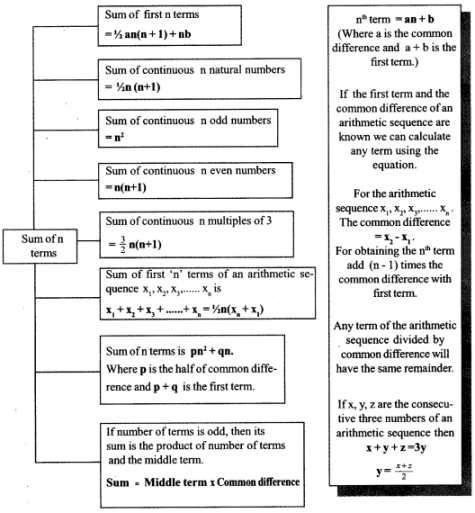# Kerala Syllabus 10th Standard Maths Solutions Chapter 1 Arithmetic Sequences

## Kerala State Syllabus 10th Standard Maths Solutions Chapter 1 Arithmetic Sequences

### Arithmetic Sequences Text Book Questions and Answers

Textbook Page No. 10

Arithmetic Sequence Questions And Answers 10th Standard Question 1.
Make the following number sequences, from the sequence of equilateral triangles, squares, regular pentagons and so on, of regular polygons:
Number of sides 3, 4, 5, ………
Sum of inner angles
Sum of outer angles
One inner angle
One outer angle
No. of triangles of a regular polygon having no. of sides are 3, 4, 5 ………… n respectively is given as 1, 2, 3, 4. Sum of interior angles of a triangle is 180°.

Then the sequence of Sum of interior angles
= 180, 180 × 2, 180 × 3 …. = 180, 360, 540, ……………….
Sum of exterior angles of any geometric structure having any number of sides is always 360°.
Sum of exterior angles = 360, 360, …………..
One interior angle
= $$\frac { 360 }{ 3 }$$, $$\frac { 360 }{ 4 }$$, $$\frac { 360 }{ 5 }$$, ……………. = 120, 90, 72, ………….

Find the sequence calculator can determine the terms (as well as the sum of all terms) of the arithmetic, geometric, or Fibonacci sequence.

Arithmetic Sequence Class 10 Kerala Syllabus Question 2.
Look at these triangles made with dots. How many dots are there in each ?Compute the number of dots needed to make the next three triangles.
3, 6, 10
The number of dots needed to make the next three triangles will be:
10 + 5 = 15
15 + 6 = 21
21 + 7 = 28
15, 21, 28 Dots

Arithmetic Sequence Question 3.
Write down the sequence of natural numbers leaving remainder 1 on division by 3 and the sequence of natural numbers leaving remainder 2 on division by 3.
The numbers that leave 1 as remainder when divided by 3 are 1, 4, 7, 10, 13, ………..
(Sequence each with difference of 3 and starting from 1)
The numbers that leave 2 as remainder when divided by 3 are 2, 5, 8, 11, 14, ………….
( Sequence each with difference of 3 and starting from 2)

Kerala Syllabus 10th Standard Maths Chapter 1 Question 4.
Write down the sequence of natural numbers ending in 1 or 6 and describe it in two other ways.
1, 6, 11, 16, 21, ……………..
Numbers, each with difference of 5 and starting from 1.
Numbers, when divided by 5, leaves 1 as remainder.

SSLC Maths Arithmetic Sequence Question 5.
A tank contains 1000 litres of water and it flows out at the rate of 5 litres per second. How much water is there in the tank after each second? Write their numbers as a sequence.
Water in the tank initially = 1000 litre
Water in the tank after first second
= 1000 – 5 = 995 litre
Water in the tank after next second
= 995 – 5 = 990 litre
Water in the tank after third second
= 990 – 5 = 9.85 litre
Sequence 1000, 995, 990, 985, 980, ………………

Textbook Page No. 15

Sslc Maths Chapter 1 Question Answer Question 1.
Write the algebraic expression for each of the sequences below:
i. Sequence of odd numbers
ii. Sequence of natural numbers which leave remainder 1 on division by 3.
iii. The sequence of natural numbers ending in 1.
iv. The sequence of natural numbers ending in 1 or 6.
i. Sequence of odd numbers = 1, 3, 5, 7 …………
That is 2 × 1 – 1,2 × 2 – 1,
Common difference a = 2,
First term, a + b=1, b = 1
Algebraic expression xn = an + b = 2n – 1
n=1, 2, 3 ………..

ii. Sequence of natural numbers which leave remainder 1 on division by 3.
1, 4, 7, 10, 13, 16
x1 = 1
x2 = 1 + 3(1) = 4
x3 = 1 + 3(2) = 7
………………………
……………………..
xn= 1 + 3(n – l) = 3n – 2
Algebraic expression xn = 3n – 2
n = 1, 2, 3 …………..

iii. The sequence of natural numbers ending in 1 is 1, 11, 21, 31……….
That is 10 × 1 – 9, 10 × 2 – 9, ……………….
Algebraic expression xn = 10n – 9
n = 1,2,3 ………….

iv. The sequence of natural numbers ending in 1 or 6 is 1, 6, 11, 16, 21 ………….
That is 5 × 1 – 4, 5 × 2 – 4,
Algebraic expression xn = 5n – 4
n=1,2,3 ……………….

An arithmetic common difference calculator is an online free tool to find the arithmetic sequence with the given common difference.

Arithmetic Sequence Pictures Question 2.
For the sequence of regular polygons starting with an equilateral triangle, write the algebraic expressions for the sequence of the sums of inner angles, the sums of the outer angles, the measures of an inner angle, and the measure of an outer angle.
Let n be the number of sides
Sum of interior angles: 180°, 360°, 540°, 720°, ……………
Algebraic expression xn = 180n
Sum of exterior angles 360°, 360°, 360°,………..
Algebraic expression xn = 360°
Sequence of one interior angle:
$$\frac { 180° }{ 3 }$$, $$\frac { 360° }{ 4 }$$, $$\frac { 540° }{ 5 }$$ ………………
Algebraic expression xn = $$\frac{180^{0} n}{n+2}$$
Sequence of one exterior angle:
$$\frac { 360° }{ 3 }$$, $$\frac { 360° }{ 4 }$$, $$\frac { 360° }{ 5 }$$, …………..
Algebraic expression xn = $$=\frac{360^{0}}{n+2}$$

Class 10 Maths Chapter 1 Kerala Syllabus Question 3.
Look at these pictures:The first picture is got by removing the small triangle formed by joining the midpoints of an equilateral triangle. The second picture is got by removing such a middle triangle from each of the red triangles of the first picture. The third picture shows the same thing done on the second.
i. How many red triangles are there in each picture?
ii. Taking the area of the original uncut triangle as 1, compute the area of a small triangle in each picture.
iii. What is the total area of all the red triangles in each picture?
iv. Write the algebraic expressions for these three sequences obtained by continuing this process.
i. The red triangles in the first picture = 3
Red triangles in the second picture = 9
Red triangles in the third picture = 27

ii. Area of the original uncut triangle = 1
Area of the first triangle = $$\frac { 1 }{ 4 }$$
Area of red triangles in the second picture = $$\frac { 1 }{ 16 }$$
Area of red triangles in the third picture = $$\frac { 1 }{ 64 }$$

iii. Total area of red triangle in the first picture = = $$3 \times \frac{1}{4}=\frac{3}{4}$$
Total area of red triangles in the second picture = $$9 \times \frac{1}{16}=\frac{9}{16}$$
Total area of red triangles in the third picture = $$27 \times \frac{1}{64}=\frac{27}{64}$$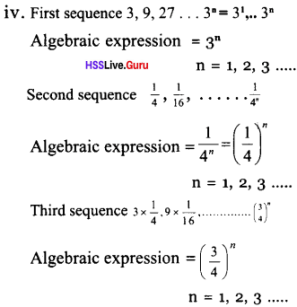This free Recursive sequence formula calculator can determine the terms (as well as the sum of all terms) of the arithmetic, geometric, or Fibonacci sequence.

Textbook Page No. 18

Arithmetic Sequence Class 10 SCERT Question 1.
Check whether each of the sequences given below is an arithmetic sequence. Give reasons. For the arithmetic sequences, write the common difference also.
i. Sequence of odd numbers.
ii. Sequence of even numbers.
iii. Sequence of fractions got as half the odd numbers.
iv. Sequence of powers of 2.
V. Sequence of reciprocals of natural numbers.
i. Sequence of odd numbers 1, 3, 5, 7,…….
Difference of adjacent numbers = 2
Common difference = 2

ii. Sequence of even numbers 2, 4, 6, 8,…….
Difference of adjacent numbers = 2 Common difference = 2
∴ This is an arithmetic sequence.

iii. Sequence of half of the odd numbers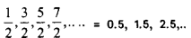Difference of adjacent numbers = 1
Common difference = $$\frac{3}{2}-\frac{1}{2}=1=\frac{5}{2}-\frac{3}{2}$$
∴ This is an arithmetic sequence.

iv. Sequence of powers of 2 21, 22, 23, 24,
2, 4, 8,16, ………………
Difference of adjacent numbers = 4 – 2 ≠ 8 – 4
It does not have a common difference
∴ This is not an arithmetic sequence

v. Sequence of reciprocals of natural numbersIt does not have a common difference
∴ This is not an arithmetic sequence

SSLC Maths Chapter 1 Question 2.
Look at these pictures: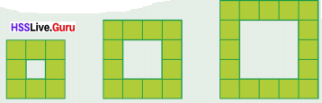If the pattern is continued, do the numbers of coloured squares form an arithmetic sequence? Give reasons.
Numbers of coloured squares in each picture 8, 12, 16, ……………
Common difference =12 – 8 = 4 = 16 – 12
∴ This is an arithmetic sequence

10th Class Maths Chapter 1 Arithmetic Sequence Question 3.
See the pictures below:i. How many small squares are there in each rectangle?
ii. How many large squares?
iii. How many squares in all?
Continuing this pattern, is each such sequence of numbers, an arithmetic sequence?
i. No., of small squares in the first picture = 2
No. of small squares in the second picture = 4
No. of small squares in the third picture = 6
No. of small squares in the fourth picture = 8

ii. No. of big squares in first picture = 0
No. of big squares in second picture = 1
No. of big squares in third picture = 2
No. of big squares in fourth picture = 3

iii. Total squares in first picture = 2
Total squares in second picture = 5
Total squares in third picture = 8
Total squares in fourth picture = 11
Sequence of no. of small squares 2, 4, 6, 8
This is an arithmetic sequence with common difference = 2
Sequence of no. of big squares, 0, 1, 2, 3
This is an arithmetic sequence with common difference = 1
Sequence of Total no. of squares 2, 5, 8, 11
This is an arithmetic sequence with common difference = 3

Question 4.
In the staircase shown here the height of the first step is 10 centimetres and the height of each step after it is 17.5 centimetres.
i. How high from the ground would be some-one climbing up, after each step?
ii. Write these numbers as a sequence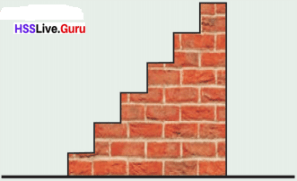i. High from the ground climbing up first step = 10 cm
High from the ground climbing up sec-ond step = 10 + 17.5 = 27.5cm
High from the ground climbing up third step = 27. 5+ 17.5 = 45 cm
High from the ground climbing up fourth step = 45 + 17.5 = 62.5 cm
High from the ground climbing up fourth step = 62.5 + 17.5 = 80 cm
High from the ground climbing up fourth ‘ step = 80 + 17.5 = 97.5cm

ii. Sequence of height
10, 27.5, 45, 62.5, 80, 97.5 ……………

Question 5.
In this picture, the perpendiculars to the bottom line are equally spaced. Prove that, continuing like this, the lengths of perpendiculars form an arithmetic sequence.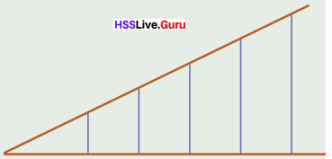In figure ΔOAB, ΔOCD are similar right-angled triangles. That is if one side and its included angles of a triangle are equal to the one side and included angles of another triangle, then the triangles are similar.
∴ sides are proportional.
$$\frac{O A}{O C}=\frac{x}{2 x}=\frac{A B}{C D} \quad \Rightarrow C D=2 A B$$ similarly
EF = 3AB. Sequence of length of perpendicular AB, 2AB, 3AB,………..
That is AB is the common difference, so perpendicular lengths are in arithmetic sequence

Question 6.
The algebraic expression of a sequence is xn = n3 – 6n2 + 13n – 7
Is it an arithmetic sequence?
Algebraic expression = n3 – 6n2 + 13n – 7
n = 1 ⇒ =1 – 6 + 13 – 7 = 1
n = 2 ⇒ = 8 – 24 + 26 – 7 = 3
n = 3 ⇒ =27 – 6 × 9 + 39 – 7
= 27 – 54 + 32 = 5
n = 4 ⇒ = 64 – 96 + 52 – 7 = 13
1, 3, 5, 13,………. This is not an arithmetic sequence.lt does not have a common difference

Textbook Page No. 21

Question 1.
In each of the arithmetic sequences below, some terms are missing and their positions are marked with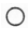. Find them.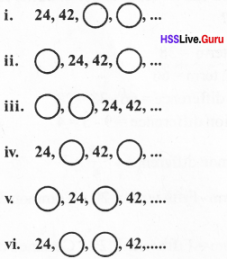Common difference = 42 – 24 = 18
Numbers to be found out = 42 + 18 = 60
60 + 18 = 78
Arithmetic sequence 24, 42, 60, 78, ………….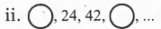Common difference = 42 – 24 = 18
Numbers to be found out = 24 – 18 = 6
42 + 18 = 60
24 – 18, 24, 42, 42 + 18
Arithmetic sequence 6, 24, 42, 60, ………….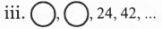Common difference = 18
Numbers to be found out = 24 – 18 = 6
6 – 18 = – 12 6 – 18, 24 – 18, 24, 42
Arithmetic sequence – 12, 6, 24, 42, ………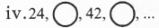Common difference = 9
[(42 – 24)/2 = 18/2 = 9)
Numbers to be found out =
24 + 9 = 33, 33 + 9 = 42, 42 + 9 = 51
Arithmetic sequence 24, 33, 42, 51 ……..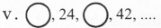Common difference = (42 + 24)/2 = 18/2 = 9
Numbers to be found out = 24 – 9, 24, 24 +9, 42
Arithmetic sequence 15, 24, 33, 42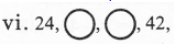Let numbers to be found out be x and y If common differences are equal = x – 24 = 42 – y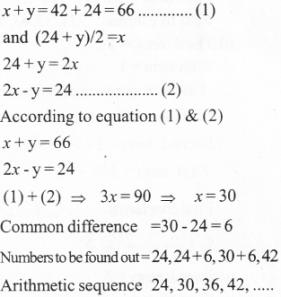Question 2.
The terms in two positions of some arithmetic sequences are given below.
Write the first five terms of each:
i. 3rd term 34
6th term 67
ii. 3rd term 43
6th term 76
iii. 3rd term 2
5th term 3
iv. 4th term 2
7th term 3
v. 2nd term 5
5th term 2
i. Third term =34
Sixth term =67
We get the 6th term from the 3 rd term, we must add the common difference (6 – 3 = 3)3 time.
Three times of common difference 67 – 34 = 33
Common difference = $$\frac { 33 }{ 3 }$$ = 11
Second term = 34 – 11 = 23
First term = 23 – 11 = 12
First five terms 12, 23, 34, 45, 56

ii. Third term =43
Sixth term = 76
Thrice the common difference = 76 – 43 = 33
Common difference = $$\frac { 33 }{ 3 }$$ = 11
Second term = 43 – 11 = 32
First term =32 – 11 =21
First five terms 21, 32, 43, 54, 65.

iii. Third term = 2
Fifth term = 3
Twice the common difference= 3 – 2 = 1
Common difference = 1/2
Second term =2 – 1/2 = 1 1/2
First term = 1 1/2 – 1/2 = 1iv. Fourth term =2
Seventh term = 3
Thrice the common difference = 3 – 2 = 1
Common difference = 1/3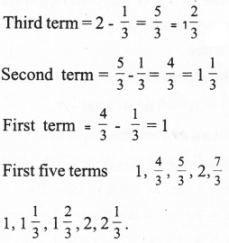v. Second term = 5
Fifth term = 2
Thrice the common difference = 2 – 5 = -3
Common difference = –3/3 = –1
First term = 5 – –1 = 6
First five terms 6, 5, 4, 3, 2

Question 3.
The 5th term of an arithmetic sequence is 38 and the 9th term is 66. What is its 25th term?
Fifth term = 38
Ninth term = 66
Term difference = 66 – 38 = 28
Position difference = 9 – 5 = 4 – 2 8
Common difference = 28/4 = 7
25th term = Fifth term = 20 × Common difference
25th term = Fifth term + 20 × Common difference = 38 + 20 × 7 = 38 + 140 = 178

Question 4.
Is 101 a term of the arithmetic sequence 13, 24, 35, …? What about 1001?
13, 24, 35,………..
Common difference = 24 – 13 = 11
Difference between 101 and 13
= 101 – 13 = 88 = 8 × 11
That is we can obtained 101 by adding 8 times of common difference with 13.
So, ‘101’ is the 9th term of this arithmetic sequence
101 is the 9th term of this sequence
Difference between 1001 and 13
= 1001 – 13 = 988
This is not a multiple of 11.
Therefore ‘1oo1’ is not a term of this arithmetic sequence.

Question 5.
How many three-digit numbers are there, which leave a remainder 3 on division by 7?
101, 108, …………………………. 997
First-term = 101
Common difference = 7
Last term = 997
Term difference = 997 – 101 = 896 = 128 × 7
Last term = First term + 128 × common difference
i. e., 997 is the 129th term.
129 three-digit numbers are there which leave remainder 3 on division by 7

Question 6.
Fill up the empty cells of the given square such that the numbers in each row and column form arithmetic sequences:What if we use some other numbers instead of 1, 4, 28 and 7?
1. In the first row differences between 1st and 4th terrm =3
Position difference = 4 – 1 = 3
Common difference = 3/3 = 1
Arithmetic sequence 1, 2, 3,4

2. In the first coloumn Term difference = 7 – 1 = 6
Position difference = 4 – 1 = 3
Common difference = 6/3 = 2
Arithmetic sequence 1, 3, 5, 7……….

3. In the fourth row
Termdifference = 28 – 7 = 21
Position difference = 4 – 1=3
Common difference = 21/3 = 7
Arithmetic sequence 7, 14, 21, 28

4. In the second coloumn 2, –,– 14
Term difference = 14 – 2 = 12
Position difference = 4 – 1=3
Common difference = $$\frac { 14 – 2 }{ 3 }$$ = 4
Arithmetic sequence 2, 6, 10, 14

5. In the third coloumn 3, –, –, 21
Term difference = 21 – 3 = 18
Position difference = 4 – 1=3
Common difference = $$\frac { 21 – 3 }{ 3 }$$ = 6
Arithmetic sequence 3, 9,15, 21

6. In the fourth coloumn 4, –, –, 28
Term difference = 28 – 4 = 24
Position difference = 4 – 1=3
Common difference = $$\frac { 28 – 4 }{ 3 }$$ = 8
Arithmetic sequence 4,12, 20, 28Question 7.
In the table below, some arithmetic sequences are given with two numbers against each. Check whether each belongs to the sequence or not.i. Sequence 11, 22, 33, …………..
Difference = 22 – 11 = 11
Difference between 123 and 11.
=123 – 11 = 112
112 is not a multiple of 11, so 123 is not a term of this arithmetic series.

Difference between 132 and 11
= 132 – 11 = 121
121 is a multiple of 11, so 132 is a term of this arithmetic series.

ii. Sequence 12, 23, 34,
Common difference = 23 – 12 = 11 Difference between 100 and 12
= 100 – 12 = 88
88 is a multiple of 11, so 100 is a term of this arithmetic series.

Difference between 1000 and 12.
= 1000 – 12 = 988
988 is not a multiple of 11, so 1000 is not a term of this arithmetic series.

iii. Sequence 21, 32, 43, ……………
Common difference = 32 – 21 = 11
Difference between 100 and 21.
= 100 – 21 = 79
79 is not a multiple of 11, so 1000 is not a term of this arithmetic series.

Difference between 1000 and 21
=1000 – 21 = 979
979 is a multiple of 11, so 1000 is a term of this arithmetic series.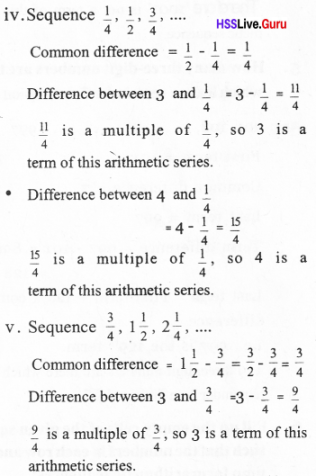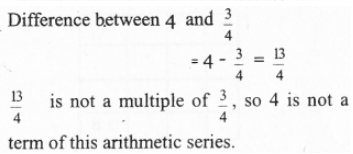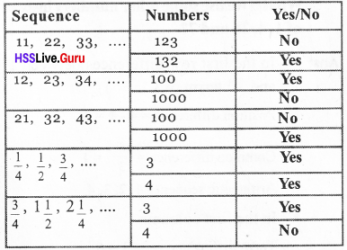Textbook Page No. 24

Question 1.
The 8th term of an arithmetic sequence is 12 and its 12th term is 8. What is the algebraic expression for this sequence?
Difference between terms = 8 – 12 = -4
To get the 12th term from the 8td term, we must add the common difference (9 – 5 = 4) 4 times.
Common difference = – 4 / 4 = -1
Algebraic expression for arithmetic series
xn = an + b
12 = – 1 × 8 + b ⇒ 12 = – 8 + b
b = 12 + 8 = 20
Algebraic expression for arithmetic series =
xn = –1 × n + 20 = 20 – n

Question 2.
The Bird problem in Class 8 (The lesson, Equations) can be slightly changed as follows.
One bird said:
“We and we again, together with half of us and half of that, and one more is a natural number”
Write all the possible number of birds starting from the least. For each of these, write the sum told by the bird also.
Find the algebraic expression for these two sequences.
If we take x as the number of birds∴ 11x + 4 is a multiple of 4.
⇒ 11x is a multiple of 4.
x is a multiple of 4.
No. of birds 4, 8, 12, 16 ……………
(a= 4, a+b = 4, b = 0)
Algebraic expression of the series = an + b = 4n No. of sums
$$\left(\frac{11 x}{4}+1, x=4,8,12 \ldots .\right)=12,23,34,45, \ldots \ldots$$
Algebraic expression for the series = 12 + (n -1)11 = 11 n + 1

Question 3.
Prove that the arithmetic sequence with first term 1/3 and common difference 1/6 contains all natural numbers.
f = $$\frac { 1 }{ 3 }$$
d = $$\frac { 1 }{ 6 }$$
$$x_{n}=d n+(f-d)=\frac{1}{6} n+\frac{1}{6}=\frac{1}{6}(n+1)$$
As n = 5, 11, 17,………….
∴ All natural numbers will occur in this arithmetic sequence

Question 4.
Prove that the arithmetic sequence with first term 1/3 and common difference 2/3 contains all odd numbers, but no even number.Value of (2n -1) is always an odd number, Therefore there is no even number.

Question 5.
Prove that the squares of all the terms of the arithmetic sequence 4,7,10,…. belong to the sequence.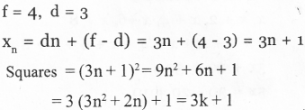This is in the form 3k + 1, so squares of all terms are in this series. (When we divide 3k + 1 by 3 get 1 as remainder)

Question 6.
Prove that the arithmetic sequence 5,8,11,……. contains no perfect squares.
f = 5
d = 3
$$x_{n}=d n+(f-d)=3 n+(5-3)=3 n+2$$
That is when we divide a perfect square with 3 we get remainder as 1 or 0. Here we can divide 3k + 2 by 3 to get 2 as remainder and it doesn’t contain any perfect square.

Question 7.
Write the whole numbers in the arithmetic sequence $$\frac { 11 }{ 8 }$$, $$\frac { 14 }{ 8 }$$, $$\frac { 17 }{ 8 }$$, ………..
they form an arithmetic sequence?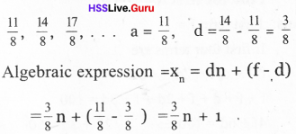If ‘n’ is a multiple of 8 then 4, 7, 10, … is a sequence having whole numbers
Common difference = 3,This is an arithmetic sequence.

Textbook Page No. 28

Question 1.
Write three arithmetic sequences with 30 as the sum of the first five terms.
If the five terms are
x, x + 1, x + 2, x +3, x + 4
x + x+ 1 + x + 2 + x + 3 + x + 4 = 30
5x + 1o = 30
5x = 20
x = 4
The arithmetic sequence is 4, 5, 6, 7, 8

If the five terms are
x, x + 2, x + 4, x +6, x + 8
x + x + 2 + x + 4 + x + 6 + x + 8 = 30
5x + 20 = 30
5x = 30 – 20 = 10
x = 2
The arithmetic sequence is 2, 4, 6, 8, 10

If the five terms are
x, x + 3, x + 6, x + 9, x + 12
x + x + 3+ x+ 6 + x + 9+ x + 12 = 30
5X + 30 = 30
5x = 0
x = 0
The arithmetic sequence is 0, 3, 6, 9,12
The arithmetic sequences whose sum of first five terms give 30 are:
4, 5, 6, 7, 8, …………..
2, 4, 6, 8, 10, …………..
0, 3, 6, 9, 12, ………….

Arithmetic Sequence Question 2.
The first term of an arithmetic sequence is 1 and the sum of the first four terms is 100.
Find the first four terms.
First term f = 1
If first four terms are
f, f+d, f+2d, f+3d
f + f + d + f + 2d + f + 3d = 100
4f + 6d = 100
= 2f + 3d = 50
2+3d = 50
= 3d = 50 – 2 = 48
d = 16
The arithmetic sequence is 1, 17, 33, 49, ………….

Question 3.
Prove that for any four consecutive terms of an arithmetic sequence, the sum of the two terms on the two ends and the sum of the two terms in the middle are the same.
First four terms of an arithmetic sequence is x,
x+ d, x+ 2d, x+ 3d
Sum of the two terms on the two ends = 2x + 3d
Sum of the two terms in the middle
= (x + d) +(x + 2d) = 2x + 3d
They both are equal

Question 4.
Write four arithmetic sequences with 100 as the sum of the first four terms.
If the first four terms are
x – 3d, x – d, x + d, x + 3d
x – 3d + x – d + x + d + x + 3d = 100
4x = 100
x = 25
i. If d= 1
The arithmetic sequence is 22, 24, 26, 28

ii. If d= 2
The arithmetic sequence is 19, 23, 27, 31

iii. If d = 3
The arithmetic sequence is 16, 22, 28, 34

iv. If d = 4
The arithmetic sequence is 13, 21, 29, 37

Question 5.
Write the first three terms of each of the arithmetic sequences described below:
i. First-term 30; the sum of the first three terms is 300.
ii. First-term 30; the sum of the first four terms is 300.
iii. First-term 30; the sum of the first five terms is 300.
iv. First-term 30; the sum of the first six terms is 300.
i. First-term = 30
Sum of first three terms = 300
In the arithmetic sequence sum of any three consecutive natural numbers is thrice the middle number
∴ Second term = $$\frac { 300 }{ 3 }$$ = 100
∴ Common difference = 300 – 30 = 270
∴ Third term =100 + 70 = 170
Sequence 30, 100, 170, ……….

ii. First-term = 30
Sum of first four terms = 300
Four consecutive terms of an arithmetic sequence, the sums of the first and the last is equal to the sum of the second and the third.
First term + Fourth term = Second term + Third term = $$\frac { 300 }{ 2 }$$ = 150
∴ Fourth term = 150 – 30 = 120
30, ………, ………., 120
Term difference = 120 – 30 = 90
Position difference = 4 – 1 = 3
Common difference = 90/3 = 30
∴ Sequence = 30, 60, 90, 120,………….

iii. First term = 30
Sum of first five terms = 300
Sum of the five consecutive terms of arithmetic sequence is five times of its middle term.
Third term = 300/5 = 60
Common difference = 15
∴ Sequence = 30, 45, 60, 75, 90, ……….

iv. First term =30
Sum of first six terms =300
Fist term + Sixth term = Second term + Fifth term = Third term + Fourth term = 300/3 = 100
Sixth term = 100 – First term = 100 – 30 = 70
Term difference = 70 – 30 = 40
Position difference =6 – 1 = 5
Common difference = $$\frac { 40 }{ 5 }$$ = 8
Sequence = 30, 38, 46, 54, 62, 70,

Question 6.
The sum of the first five terms of an arithmetic sequence is 150 and the sum of the first ten terms is 550.
i. What is the third term of the sequence?
ii. What is the eighth term?
iii. What are the first three terms of the sequence?
i. Sum of first five terms = 150
Sum of the five consecutive terms of arithmetic sequence is five times of its middle term.
Third term = $$\frac { 150 }{ 5 }$$ = 30

ii. First term + Tenth term = Second term + Nineth term = Third term + Eighth term = Fourth term + Seventh term = Fifth term + Sixth term = $$\frac { 550 }{ 5 }$$ = 110
Third term + Eighth term = 110
Eighth term =110 – Third term
= 110 – 30 = 80

iii. Third term = 30
Eighth term = 80
Term difference = 80 – 30 = 50
Position difference = 8 – 3 = 5
Common difference = $$\frac { 50 }{ 5 }$$ = 10
∴ Sequence = 10, 20, 30,…………….

Question 7.
The angles of a pentagon are in arithmetic sequence. Prove that its smallest angle is greater than 36°.
Let the smallest angle in the pentagon be x
x + x + d + x + 2d + x + 3d + x + 4d = 540
5x + 10d = 540,
x + 2d = 108
x = 36°, then
d = 36,
angles are 36, 72, 108, 144, 180.
180° will not an angle of a pentagon (Sum of exterior and interior angle is 180°).
Therefore the smallest angle in a pentagon will always be greater than 36°

Textbook Page No. 35

Question 1.
Find the sum of the first 25 terms of each of the arithmetic sequences below.
i. 11, 22, 33,….
ii. 12, 23, 34,…
iii. 21, 32, 43,….
iv. 19, 28, 37,…
vi. 1, 6, 11,….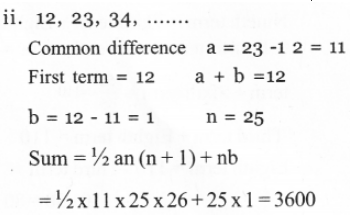Question 2.
What is the difference between the sum of the first 20 terms and the next 20 terms of the arithmetic sequence 6, 10, 14,…?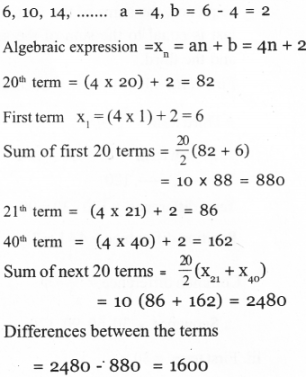Differences = 2480 – 880 = 1600

Question 3.
Calculate the difference between the sums of the first 20 terms of the arithmetic sequences 6, 10, 14,… and 15, 19, 23,……
6, 10, 14,
Algebraic expression of the above arithmetic sequence = 4n + 2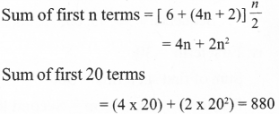15, 19, 23, …… Algebraic expression of the arithmetic sequence = 4n +11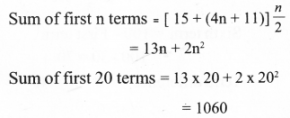Differences = 1060 – 880 = 180

Question 4.
Find the sum of all three-digit numbers, which are multiples of 9.
First 3 digit number divisible by 9 = 108
Last 3 digit number divisible by 9 = 999 108, 117,126, …………… 999Question 5.
The expressions for the sum to n terms of some arithmetic sequences are given below. Find the expression for the nth term of each:
i. n2 + 2n
ii. 2n2 + n
iii.n2 – 2n
iv.2n2 – n
v. n2 – n
i. n2 + 2n, First term =12+ 2 × 1 = 3
Sum of first two terms = 22 + 2 × 2 = 8
Second term = 8 – 3 = 5
The arithmetic sequence is 3, 5,…………..
nth term = dn + (f – d) = 2n + (3 – 2)
= 2n + 1

ii. 2n2 + n
First term =2 + 1 = 3
Sum of first two terms =2 × 22 + 2
= 8 + 2 = 10
Second term = 10 – 3 =7
The arithmetic sequence is 3, 7, …………iii. n2 – 2n
First term = 1 – 2 = – 1
Sum of first two terms =22 – 4 = o
Second term = 0-(-1) = 0 + 1 = 1
The arithmetic sequence is –1, 1, ……………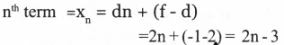iv. 2n2 – n
First term = 2 – 1 = 1 Sum of first two terms = 2 – 22 – 2
=8 – 2=6
Second term = 6 – 1 = 5
The arithmetic sequence is 1, 5,……….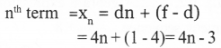v. n2 – n
First term = 1 – 1 = 0
Sum of first two terms = 22 – 2 = 2
Second term = 2 – 0 = 2
The arithmetic sequence is 0, 2,……….Question 6.
Calculate in head, the sums of the following arithmetic sequences.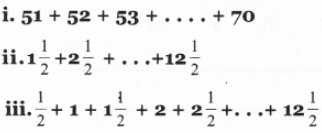Question 7.
Prove that the sum of any number of terms of the arithmetic sequence 16, 24, 32,….. starting from the first, added to 9 gives a perfect square
16, 24, 32,…………. Algebraic expression of the arithmetic sequence = 8n + 8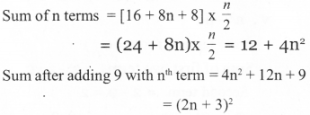Hence it is a perfect square

Question 8.i. Write the next two lines of the pattern above.
ii. Write the first and the last numbers of the 10th line.
iii. Find the sum of all the numbers in the first ten lines.
i. First row contains one element, second row contains two elements, therefore j fifth row will contain five elements and j sixth row will contain six elements.
Consider the first row
1, 2, 4, …………..
1, 1+1, 2 + 2, 4 + 3, …………
Therefore
1, 1+1, 2 + 2, 4 + 3, 7 + 4, 11+5 …………..
1, 2, 4, 7, 11, 16 …………
Generally it written as
1 + 1 (1+2 + 3 + ……….)
Fist term in the fifth row = 1 + 1(1 + 2 + 3 + 4) = 1 + 10 = 11
Fist term in the sixth row= 1 + 1(1 + 2 + 3 + 4+ 5) = 1 + 15 = 16
Common differences in each row = 1
Fifth row 11, 12, 13, 14, 15.
Sixthrow 16, 17, 18, 19, 20, 21.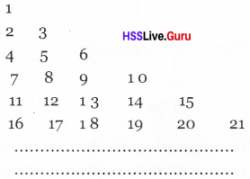ii. Fist term in the tenth row = 1 + 1(1 + 2 + 3+ 4 + 5 + 6+ 7 + 8 + 9) = $$=1+\frac{1 \times 9 \times 10}{2}=46$$
Last term in the tenth row = 46 + 1 × 9 = 46 + 9 = 55

iii. Numbers in the first ten lines 1, 2, 3, 4, 5, ………….. 55
Sum of all the numbers in the first ten lines = $$\frac{n(n+1)}{2}=\frac{55 \times 56}{2}=1540$$

Question 9.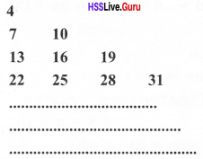Write the next two lines of the pattern above. Calculate the first and last terms of the 20th line.
4, 7, 13, 22, 34, 49,…….
(4, 4+3, 7+6, 13+9, 22 + 12, 34+15),
Generally it written as 4 + 3(1 + 2 + 3 + ………..)
First term in the fifth row = 4 + 3(1 +2 + 3+ 4) = 4 + 30 = 34
First term in the sixth row =
4 + 3(1 + 2+ 3 + 4 + 5) = 4 + 45 = 49
Common differences in each row = 3
Fifth row 34, 37, 40, 43, 46,..
Sixth row 49, 52, 55, 58, 61, 64…
4
7 10
13 16 19
22 25 28 31
34 37 40 43 46
49 52 55 58 61 64
………………………….
First term in the 20th row = 4 + 3(1 + 2 + 3 + 4 +……………. +19)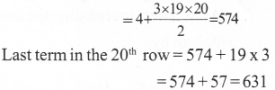### Arithmetic Sequences Orukkam Questions & Answers

Worksheet 1

Question 1.
Write the sequence of natural numbers
1,2,3,4,

Question 2.
Write the sequence of odd numbers
1,3,5,7,

Question 3.
Write the sequence of even numbers
2,4,6,8,10,

Question 4.
Write the sequence of multiples of 3.
3,6,9,12,15,

Question 5.
Write the sequence of numbers which leaves the remainder 1 on dividing by 4.
0 × 4 + 1, 1 × 4 + 1, 2 × 4 + 1, 3 × 4 + 1, 4 × 4 + 1, 5 × 4 + 1, …………
=1, 5, 9, 13, 17, 21,

Question 6.
Write the sequence of prime numbers.
2,3,5,7,11,13,17,

Question 7.
Write the sequence of perfect squares.
1, 4, 9, 16, 25, 36,

Question 8.
Write the sequence of numbers which leaves the remainder 0 on dividing by 6
6, 12, 18, 24, 30, 36, ………….

Question 9.
Write the sequence starting from 1 and is added subsequently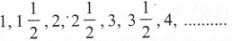Question 10.
Write the sequence starting from 1/2 and 3/4 is added subsequently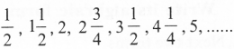Question 11.
Write the sequence starting from 60 and 0 is added subsequently
60, 60, 60,…………..

Worksheet 2

Question 12.
Write the sequence of the perimeters of the equilateral triangles having sides 1cm, 2cm, 3cm.
a. Write the sequence of area
b. Write the sequence of sum of angles.
’If we Increase 1 cm of sides of an equilateral triangle having sides 1cm, 2cm, 3cm.
a. Area =1 cm2, Area after increasing
sides by 1 cm $$\frac{\sqrt{3} \times 2^{2}}{4}=\sqrt{3}$$ cm2
Area after increasing thelength of side 2cm by
V3x32 9V3 ,
1cm = $$=\frac{\sqrt{3} \times 3^{2}}{4}=\frac{9 \sqrt{3}}{4}$$ cm2
Area after increasing thelength of side 3cm by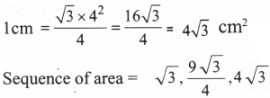c. The sum of angles of a triangle will be 180° always for any measures of sides. Sequence of sum of angles 180, 180, 180,……..

Question 13.
Write the sequence of numbers which leaves the remainder 3 on dividing by 5 and 10.
The number which can be divided by 5 and 10 must be divisible by 10.
∴ The sequence of numbers which leaves the remainder 3 on dividing by 5 and 10 is 3, 13, 23, 43,………

Question 14.
Look at the sequence 1 + (1 + 5), 2 + (2 + 5), 3 + (3 + 5) …….
a. Write next two terms
b. Write its algbraic form.
a. Next two terms
4 + (4 + 5), 5 + (5 + 5),

b. Algebraic expression
xn = n + (n + 5) = n + n + 5
xn = 2n + 5

Question 15.
Write the terms of the sequence 5 × (1+6), 10 × (2+6), 15 × (3+6), 20 × (4+6) in the form :
first term 5 × 1(1 + 6),
second term 5 × 2(2 + 6).
Write its algebraic expression
5 x (1+6), 10 x (2 + 6), 15 x (3 + 6), 20 x (4 + 6), … this sequence can be written as 5 x 1(1 + 6), 5 x 2 (2 + 6), 5 x 3 (3 + 6), 5 x 4 (4 + 6). Algebraic expression = 5 x n (n + 6)
i.e. xn = 5 n2 + 30n.

Worksheet 3

Question 16.
Write eighth terms of an arithmetic sequence using the numbers given below. (22, 15, 18, 4, 10, 14, 6, 12)
6, 10, 14, 18, 22, ……………

Question 17.
Write the missing terms in the arithmetic sequence given belowQuestion 18.
The difference between 12th and 8th term of an arithmetic sequence is 20. Find the common difference.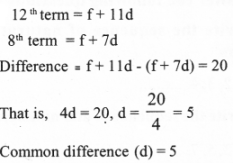Question 19.
The tenth term of an arithmetic sequence is 65 and its 15th term is 80. Is 200 a term of this sequence?
’To get the 15th term from the 10th term, we must add the common difference 5 times.
5 times of common difference = 80 – 65 = 15
Common difference (d) = 15/5 = 3
Dividing 65 by 3 we get the remainder as 2.
Dividing 200 by 3 we get the remainder as 2.
Therefore 200 is a term of this sequence.

Question 20.
The 20th term of an arithmetic sequence is 64 and its 21th term is 70. Can the difference between two terms 46? Why?
20th term = 64
21th term = 70
Common difference = 70 – 64 = 6 Difference between any two terms of this sequence will be a multiple of 6.
Dividing 46 by 6 we get the remainder as 4, So difference between any two terms of the sequence will not be 46.

Question 21.
The angles of a quadrilateral are in an arithmetic sequence. The largest angle is 150°. Find other angles.
Sum of four angles of a quadrilateral = 360° Angles are in arithmetic sequence, so first angle + 3 x common difference (d) = 150°
i.e., f + 3d= 150The four angles are f, 70, f + 2d, 150 .
$$\mathrm{f}+2 \mathrm{d}=\frac{150+70}{2}=110$$
Common difference = 110 – 70 = 40
First angle = 70 – 40 = 30
So, the four angles are 30, 70, 110, 150

Question 22.
What will be the remainder on dividing a term of the sequence 3n + 7 by its common difference?
Dividing 3n + 7 by 3 we get the remainder as 1.

Worksheet 4

Question 23.
Write the algebra of the following sequences and its sum of n terms
1.5, 10, 15, 20, ………..
2. 6, 11, 16, 21, ………
3. 4, 9,14,19, ……….
4. 3, 8, 13, 18, …………..
1. 5, 10, 15, 20,
First term f = 5
Common difference = d = 10 – 5 = 5
General form xn = dn + (f – d)
= 5n + (5 – 5) = 5n2. 6, 11, 16,21, …………..
First term f = 63. 4, 9, 14, 19, ………….
First term f = 4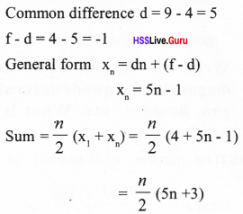4. 3, 8, 13, 18, …………
First term f = 3Worksheet 6

Question 24.
Write the sequence of the squares of all odd numbers. What is its algebra?
Odd numbers = 1, 3, 5, 7, 9, 11, ………
f= l, d = 3 – 1 =2, f – d= 1 – 2 = – 1
Algebraic form of odd numbers
xn= dn+ (f – d) = 2n – 1
The sequence of the squares of all odd numbers = 1, 9, 2 5, 49,……….
Algebraic form = (2n -1 )2

Question 25.
Write the sequence formed by the number of diagonals from a vertex of a triangle, a quadrilateral, a pentagon etc. What is its algebra?
Diagonal drawn from one vertex of a triangle = 0 = 3 – 3 = 0
Diagonal drawn from one vertex of a quadrilateral = 4 – 3 = 1
Diagonal drawn from one vertex of a pentagon = 5 – 3 = 2
Diagonal drawn from one vertex of a n sided polygon = n – 3
Sequence of number of diagonals 0, 1, 2, 3, 4, ……….
Algebraic expression xn = n – 3

Question 26.
Write the sequence of the number of diagonals in a quadrilateral, pentagon, hexagon etc. What is its algebra?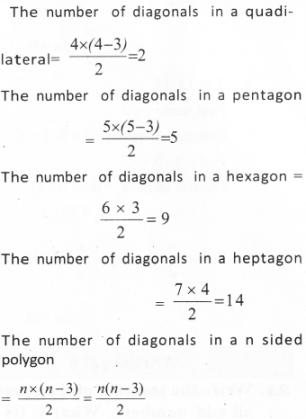Question 27.
Can the difference between any two terms of an arithemetic sequence having common difference 6 be 2016? Justify your answer.
$$\frac{\text { Differences of terms }}{\text { Common difference }}=\frac{2016}{6}=336$$
It is a natural number, so the difference between any two terms of an arithemetic sequence having common difference 6 be 2016.

Question 28.
Write algebra of the sum of the sequence 6n + 5. Can the sum 2000 ? Why?
nth term = 6n + 5
1st term = 6 + 5 = 11
Sum of n terms = n/2 (11 + 6n + 5)
= n/2 (6n + 16) = n(3n + 8) = 3n2 + 8n
Algebraic form of the sum = 3n2 + 8n
To check wheater the sum is 2ooo
3n2 + 8n = 2000
i.e., 3n2 + 8n – 2000 = 0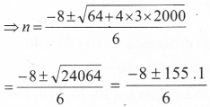It is not a natural number. So 2000 will not be a sum.

Worksheet 7

Question 29.
Prove that the squares of the sequence 1, 3, 5,……..belongs to that sequence itself.
Odd-numbered arithmetic sequence will be 1, 3, 5, …
Here the arithmetic sequence have common difference 2. Their squares are also odd numbers. Therefore the squares of the sequence 1, 3, 5, …belongs to that sequence itself. nth term = 2n – 1.

Question 30.
In an arithmetic sequence having terms natural numbers, prove that if one of the terms is a perfect square, it will have more that this as the perfect square term.
As we know when a definite number of common difference of an arithmetic sequence is added to a term we get another term of the same sequence. If n2 is a perfect square term, add (2n + d) times d to n2. n2 + (2n + d) x d = (n + d)2. This is nothing but a perfect square term.

Question 31.
If the angles of a right triangle are in an arithmetic sequence, find them by making suitable equations.
Let angles be f – d, f, f + d f – d + f + f+d = 180
3f = 180, f = 60, f + d = 90, d = 30 Angles are 30, 60 and 90

Question 32.
If ten times tenth term of an arithmetic sequence is equal to fifteen times fifteenth term, find 25th term. Calculate the product of first 25th terms.
10 × (f + 9d) = 15 × ( f + 14 d)
⇒ 10 f + 90 d = 15 f + 210 d
⇒ 5 f+ 120 d = 0
⇒ f + 24 d=0
25th term = f + 24 d = 0
25th term is 0. Product of 25 terms is 0.

Question 33.
Write the algebraic form of 1,4,7,10,… is 100 a term of this sequence. Why? Prove that the square of any term of this sequence belongs to that sequence.
1,4, 7, 10,…
f = 1, d = 3
xn= dn + (f – d) = 3n + (1 – 3) = 3n – 2
When 4 is divided by 3 we get remainder as 1 When 100 is divided by 3 we get remainder as 1 So, 100 is a term of the arithmetic sequence.
Square = (3n – 2)2 = 9n2 – 12n + 4 (9n2 – 12n + 4) + 3
When 9n2 – 12n + 4 is divided by 3the remainder is 1.
So square of the term also in the sequence.
∴ The square of any term of this sequence belongs to that sequence.

Question 34.
Write the sequence obtained by adding two adjacent consecutive terms in counting numbers starting from 1.
Write the algebraic expression of this sequence.
[Score: 3, Time: 4 minutes]
Counting numbers:
1, 2, 3, 4, 5, … (1)
: 1+2, 2 + 3, 3 + 4, 4 + 5, … (1) Two adjacent consecutive terms
3, 5, 7, 9, …
Algebraic expression of above sequence :
n + (n+l) = 2n + 1 (1)

Question 35.
Consider circles, points on its circumference and chords as shown in the figure. Mark two points on the circle and draw a chord. Mark one more point and draw three chords. Continue this process by adding one more point each time. [Score: 4, Time: 7 minutes]
a. Write the number of chords in each figure as a sequence.
b. Write the algebraic expression of this sequence.
c. Find the number of chords in the 10th figure.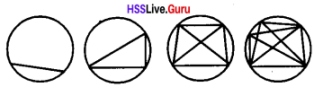a. No. of chords in figure 1 = 1
No. of chords in figure 2 = 1 + 2 = 3
No. of chords in figure 3 = 1+ 2 + 3 = 6 (1)
Sequence of number of chords
= 1, 3, 6, 10, … (1)

b. No. of chords in figure n
$$=1+2+3+\ldots+n=\frac{n(n+1)}{2}$$ (1)

c. No. of chords in the 10th figure
$$=\frac{10 \times 11}{2}=55$$ (1)

Question 36.
A pattern is formed using sticks of equal length as shown below:
[Score: 4, Time: 9 minutes]a. Write the sequence of number of sticks used in each figure.
b. Write the sequence of number of squares and rectangles in each figure,
c. Write the algebraic expression in the above two sequences.
d. Find the number of sticks and squares in the 10th figure.
a. No of sticks in figure 1 = 1 + 3 = 4
No of sticks in figure 2
= 1 + 3 + 3 = 1+2 × 3 =7 No of sticks in the figure 3
= 1 + 3 × 3 = 10 No of sticks in the figure 4
= 1+4 × 3 = 13 Sequence of number of sticks
= 4, 7, 10, 13, … (i)

b. No of squares and rectangles in the figure 1 = 1
No of squares and rectangles in the figure 2 = 2 + 1 = 3
No of squares and rectangles in the figure 3 =3 + 2+ 1=6
No ofsquares and rectangles in the figure 4 = 4 + 3 + 2 + 1 = 10
Sequence of squares and rectangles = 1, 3, 6, 10, …. (1)

c. No of sticks in the nth figure 1
= 1+ n × 3 = 3n + 1
No of squares and rectangles in the nth figure = 1 + 2+ 3….. +n = $$=\frac{n(n+1)}{2}$$ (1)

d. No of sticks in the 10th figure = 3 × 10 + 1 = 31
No of squares and rectangles in the 10th figure = $$\frac{10 \times 11}{2}=55$$ = 55 (1)

37. Consider an arithmetic sequence with common difference 6 and 7th term 52. Find the 15th term of the arithmetic sequence. Is it possible, to get a difference of 100 between any two terms of this sequence?
[Score: 3, Time: 5 minutes]
15th term can be obtained by adding 8 times the common difference to the 7th term.
x15 = x7 +8d (1)
= 52 + 8 × 6 = 100 (1)
The difference between any two terms of an Arithmetic sequence will be a multiple of com-mon difference. 100 can’t be the difference be-tween any two terms of this sequence, since it is not a multiple of 6. (1)

Question 38.
Consider an arithmetic sequence whose 7th term is 34 and 15th term is 66.
[Score: 3, Time: 5 minutes]
a. Find the common difference,
b. Find the 20th term.
a. 15th term can be obtained by adding 7th term ‘ and 8 times the common difference.
x15 = x7 + 8d (1)
66 = 34 + 8d
8d = 66 – 34 = 32
d = $$\frac { 32 }{ 8 }$$ = 4 (1)

d. 20th term can be obtained by adding 15th term and 5 times the common difference.
x20 = x15 + 8d (1)
= 66 + 5 × 4 = 86

Question 39.
Consider an arithmetic sequence $$\frac { 17 }{ 7 }$$, $$\frac { 20 }{ 7 }$$, $$\frac { 23 }{ 7 }$$, ……….
a. Write the algebraic expression of the sequence.
b. Write the sequence of counting numbers in the above given sequence. Is the newly obtained sequence an arithmetic sequence. [Score:. 4, Time: 6 minutes]
a. Common Difference = $$\frac { 20 }{ 7 }$$ – $$\frac { 17 }{ 7 }$$ = $$\frac { 3 }{ 7 }$$ (1)
Algebraic expression of the sequence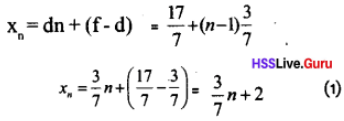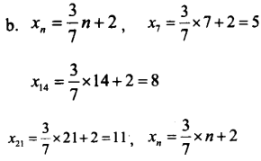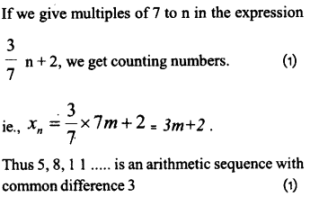Question 40.
Let the algebraic expression of an arithmetic sequence be 5n + b. If there is no perfect square in this sequence, find the counting number less than 5 that can be the value of ‘b’. [Score:4, Time: 5minutes]Any perfect square when divided by 5 leaves remainder 0, 1,4 So if remainder is 2 and 3 then it will not be a perfect square.
If there is no perfect square in the sequence, the only possibility for value of ‘b’ less than 5 is 2 and 3. (1)

### Arithmetic Sequences Exam Oriented Questions & Answers

Short Answer Type Questions (Score 2)

Question 41.
—, 18, —, 28 are four consecutive terms of an arithmetic sequence. Fill in the blanks.
18 + 2d = 28
2d = 28 – 18 = 10
d = 5
Sequence 13, 18, 23, 28.

Question 42.
98 is a term of the arithmetic sequence having common difference 7. is 2016 a term of this sequence. Why?
If (2016 – 98) is a multiple of common difference 7, then 2016 is a term of the arithmetic sequence.
2016 – 98 = 1918
1918 is a multiple of 7.
(Quotient = 274, Remainder = 0)
∴ 2016 is a term of this sequence.

Question 43.
For the arithmetic sequence 6, 12, 18,………
a. What is the common difference?
b. Find the 10th term?
a. Common difference = 6
b. 10th term = f + 9d = 6 + (9 × 6)
= 6 + 54 = 60

Question 44.
The algebraic form of an arithmetic sequence is 3 + 2n.
a What is the first form of the sequence?
b. What will be the remainder if the terms of the sequences are divided by 2?
a. Firstterm = 3 + 2 x 1 = 5
b. d = 2 (coefficient of n be the common difference)
The remainder divided by 2 = 1

Short Answer Type Questions (Score 3)

Question 45.
The nth term of an arithmetic sequence is an = 5 – 6n. Finditssumofnterms?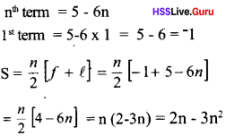Question 46.
Consider the multiples of 7 in between 100 and 500.
a. What are the first and last numbers?
b. How many terms are there in the sequence?
a. Firstteim=100 – 2 + 7 = 105
Last term = 500 – 3 = 497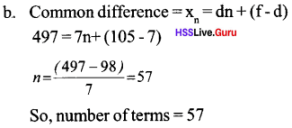Question 47.
For an arithmetic sequence 22, 26, 30, …………..
a. What is the common difference?
b. Will 50 be a term of this sequence? Why?
c. Can the difference between any two terms of this sequence be 50? Justify your answer?
a. Common differenced = 26 – 22 = 4
b. $$\frac{50-22}{4}=7$$
So, 50 is a term of this sequence,
c. 50 is not a multiple of 4. So, 50 is cannot be a difference of two terms.

Question 48.
Find the smallest 3 digit number which is the multiple of 6. Find the sum of all the three-digit numbers which are the multiple of six.
Smallest 3 digit number which is the multiple of 6 = 102,
Highest =996
Common difference = 6
Arithmetic series : 102, 108, …………….. 996
Number of three digit numbers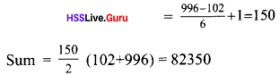Question 49.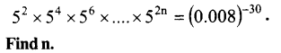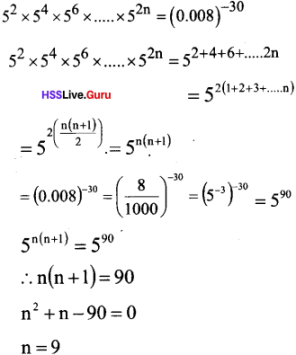Question 50.
Find the sum of first 24 terms of the list of numbers whose nth term is given by an = 3 + 2n.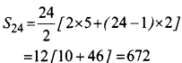Long Answer Type Questions (Score 4)

Question 51.
a. Write the arithmetic sequence with first term 2 and common difference 3.
b. Check whether 100 is a term in this sequence.
c. Check whether the difference of any two terms of this sequence will be 2015.
d. Find the position of the term 125 in this sequence.
a. f = 2
d. = 3
Sequence is 2, 5, 8, 11, ……….

b. If (100 – 2) is not a multiple of common difference 3, then 100 is not a term of the arithmetic sequence.
c. 2015 is not a multiple of common difference 3, so 2015 will not be the difference of any two terms of this sequence.

d. xn= 3n – 1
3n-1 = 125
3n= 126
n = 42

Question 52.
When 60 added to the first term of an A.P, we get its 11th term. Which number should be added to its first term to get the 19th term? Can 75 be the difference between any of the two terms of this sequence?
Differences between first term and 11th term is = 60
(11 – 1 = 10)
10 times of common difference = 60
Common difference = 60/10 = 6
Differences between first term and 19th term is = 18 x common difference = 18 x 6 = 108 When 108 is added to the first term, we get 19th
term. The difference between two terms in an A.P is the multiple of common difference. 75 is not the multiple of common difference 6. So 75 cannot be the difference between two terms in the series.

Question 53.
i. What is the sum of first 20 natural numbers?
ii. The algebraic form of an arithmetic sequence is 6n + 5. Find the sum of first 20 terms of this sequence?Question 54.
23rd term of an arithmetic sequence is 32. 35th term is 104. Then
a. What is the common difference?
b. Which is the middle term of first 35 terms of this sequence?
c. Find the sum of first 35 terms of this sequence.b. Middle term offirst 35 terms = $$\sqrt { 4 }$$
c. 18 th term = X19 = X23 – 5d = 32 -5 × 6 = 32 – 30 = 2
Sum of 35 terms = 18th term × 35 = 70

Question 55.
The difference between the 15th term and the 5th term of an A.P is 40. Which number is to be added to its 12 Answer:
The difference between the 15th term and the 5th term = 40
i.e., ten times an of a common difference = 40
Common difference = 40/10 = 4
When we add 8 x common difference we will get 20th term.
The difference between the 12th term and the 20th term = (20 – 12) × common difference
= 8 x 4 = 32
The difference between the first term and the 21th term = (21 – 1) × Common difference
= 20 × = 80

Long Answer Type Questions (Score 5)

Question 56.
Consider the arithmetic sequence 171, 167, 163,………..
i) Is ‘0’ is a term of this sequence? Why?
ii) How many positive terms are in this sequence?
Arithmetic senes : 171, 167, 163 …………….∴ 0 will not be a term of the sequenceQuestion 57.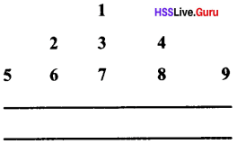a. How many numbers are there in the 30th row of this number pyramid?
b. Which is the last number in the 30th row?
c. Which is the first number in the 30th row?
d. What is the sum of all terms in the first 30 rows?
a. Total numbers in each sequence can be written as 1, 3, 5, …. xn = 2n – 1
Numbers in the 30th row = 2 x 30 – 1 = 59

b. Last number in the first row = 12 = 1
Last number in the second row = 22 = 4
Last number in the third row = 32 = 9
Last number in thew 30th row = 302 = 900

c. Number of terms in the 30th row = 59
Last number in the 30th row = 900
First number in the 30th row + 58d = Last term in the 30th row
First number in the 30th row + 58 x 1 = 900 First number in the 30th row = 900 – 58 = 842

d. The sum of all terms in the first 30 rows = $$\begin{array}{l}{\frac{900 \times 901}{2}} \\ {=450 \times 901=405540}\end{array}$$

Arithmetic Sequences Memory Map